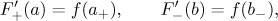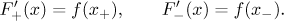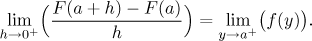Theorem (The Fundamental Theorem of Calculus, TFC)
Let f be a function on [a,b]. Assume that f is Riemann integrable on [a,b] and that it also has an antiderivative F on [a,b]. ThenIn short, this says that if a function has both the Riemann and Newton integral, they must be connected by the Newton-Leibniz formula. Note that the Riemann and Newton integrability in this general version is less restrictive than the continuity we required in the main text, so this version is more general and therefore more powerful. However, in actual problems we usually use continuity anyway because it is the easiest way out and we usually get continuous functions anyway.

Similarly, the first version of TFC we stated in the main text is true in more generality:

Theorem (The Fundamental Theorem of Calculus I, TFC 1)
Let f be a Riemann integrable function on [a,b], let c belong to [a,b]. For x from [a,b] we defineThen F is continuous on (a,b), it has derivative from the right at all points from [a,b) and derivative from the left at all points from (a,b]. Moreover,and for every x from (a,b) we haveRecall that for instance F ′+(a) denotes the right derivative of F at a, and f (a+) denotes the limit of f at a from the right. So the first statement in fact means that